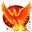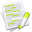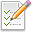#wx.lib.plot.polyobjects.PolyPoints¶

Base Class for lines and markers.

param points

The points to plot

type points

list of (x, y) pairs

param attr

type attr

dict

Warning

All methods are private.

##Class Hierarchy¶Inheritance diagram for class PolyPoints:

##Methods Summary¶

 __init__ Initialize self. See help(type(self)) for accurate signature. boundingBox Returns the bounding box for the entire dataset as a tuple with this getClosestPoint Returns the index of closest point on the curve, pointXY, getLegend scaleAndShift Scales and shifts the data for plotting. setLogScale Set to change the axes to plot Log10(values)

##Properties Summary¶

 absScale A tuple of (x_axis_is_abs, y_axis_is_abs) booleans. If a value logScale A tuple of (x_axis_is_log10, y_axis_is_log10) booleans. If a value points Get or set the plotted points. symLogScale Not yet implemented. symLogThresh Not yet implemented.

##Class API¶

class PolyPoints(object)

Base Class for lines and markers.

Parameters
• points (list of (x, y) pairs) – The points to plot

• attr (dict) – Additional attributes

Warning

All methods are private.

### Methods¶

__init__(self, points, attr)

Initialize self. See help(type(self)) for accurate signature.

boundingBox(self)

Returns the bounding box for the entire dataset as a tuple with this format:

((minX, minY), (maxX, maxY))

Returns

boundingbox

Return type

numpy array of [[minX, minY], [maxX, maxY]]

getClosestPoint(self, pntXY, pointScaled=True)

Returns the index of closest point on the curve, pointXY, scaledXY, distance x, y in user coords.

if pointScaled == True, then based on screen coords if pointScaled == False, then based on user coords

getLegend(self)

scaleAndShift(self, scale=(1, 1), shift=(0, 0))

Scales and shifts the data for plotting.

Parameters
• scale (list of floats: [x_scale, y_scale]) – The values to scale the data by.

• shift (list of floats: [x_shift, y_shift]) – The value to shift the data by. This should be in scaled units

Returns

None

setLogScale(self, logscale)

Set to change the axes to plot Log10(values)

Value must be a tuple of booleans (x_axis_bool, y_axis_bool)

Deprecated since version Feb: 27, 2016

Use the logScale property instead.

### Properties¶

absScale

A tuple of (x_axis_is_abs, y_axis_is_abs) booleans. If a value is True, then that axis is plotted on an absolute value scale.

Getter

Returns the current value of absScale

Setter

Sets the value of absScale

Type

tuple of bool, length 2

Raises

ValueError – when setting an invalid value

logScale

A tuple of (x_axis_is_log10, y_axis_is_log10) booleans. If a value is True, then that axis is plotted on a logarithmic base 10 scale.

Getter

Returns the current value of logScale

Setter

Sets the value of logScale

Type

tuple of bool, length 2

Raises

ValueError – when setting an invalid value

points

Get or set the plotted points.

Getter

Returns the current value of points, adjusting for the various scale options such as Log, Abs, or SymLog.

Setter

Sets the value of points.

Type

list of (x, y) pairs

Note

Only set unscaled points - do not perform the log, abs, or symlog adjustments yourself.

symLogScale

Warning

Not yet implemented.

A tuple of (x_axis_is_SymLog10, y_axis_is_SymLog10) booleans. If a value is True, then that axis is plotted on a symmetric logarithmic base 10 scale.

A Symmetric Log10 scale means that values can be positive and negative. Any values less than symLogThresh will be plotted on a linear scale to avoid the plot going to infinity near 0.

Getter

Returns the current value of symLogScale

Setter

Sets the value of symLogScale

Type

tuple of bool, length 2

Raises

ValueError – when setting an invalid value

symLogThresh

Warning

Not yet implemented.

A tuple of (x_thresh, y_thresh) floats that define where the plot changes to linear scale when using a symmetric log scale.

Getter

Returns the current value of symLogThresh

Setter

Sets the value of symLogThresh

Type

tuple of float, length 2

Raises

ValueError – when setting an invalid value